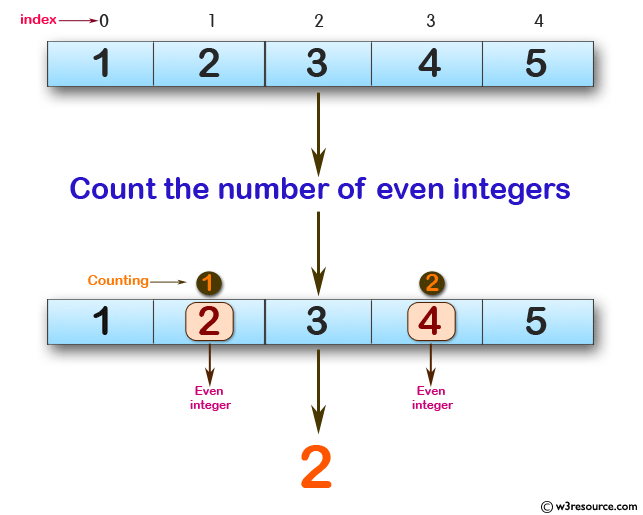﻿ Swift Array Programming Exercise: Count the number of even integers in the given array - w3resource# Swift Array Programming Exercises: Count the number of even integers in the given array

## Swift Array Programming: Exercise-24 with Solution

Write a Swift program to count the number of even integers in the given array.

Pictorial Presentation:Sample Solution:

Swift Code:

``````func countEvens(a: [Int]) -> Int {
var ctr = 0

for x in 0..<a.count {
if a[x] % 2 == 0 {
ctr += 1
}
}

return ctr
}
print(countEvens(a: [1, 2, 3, 4, 5]))
print(countEvens(a: [1, 3, 5, 7]))
print(countEvens(a: [2, 4, 6, 8]))
```
```

Sample Output:

```2
0
4
```

Swift Programming Code Editor:

Improve this sample solution and post your code through Disqus

What is the difficulty level of this exercise?

﻿